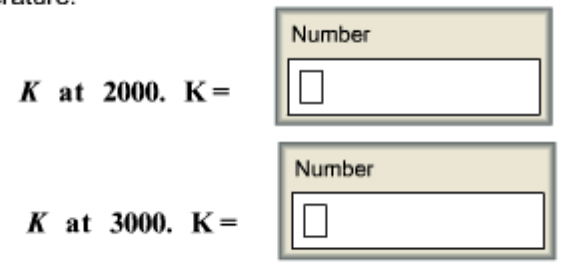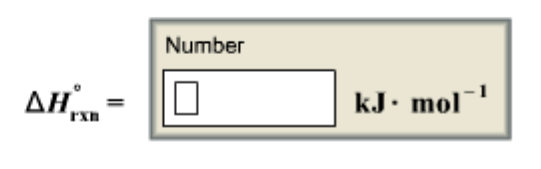# Problem: The following equation represents the decomposition of a generic diatomic element in its standard state. 1/2 X2 (g) → X (g) Assume that the standard molar Gibbs energy of formation of X (g) is 5.14 kJ • mol-1 at 2000 K and -51.10 kJ • mol-1 at 3000 K. Determine the value of K (the thermodynamic equilibrium constant) at each temperature. Assuming that ΔH°rxn is independent of temperature, determine the value of ΔH°rxn from these data.

###### FREE Expert Solution
93% (123 ratings)
###### FREE Expert Solution

93% (123 ratings)###### Problem Details

The following equation represents the decomposition of a generic diatomic element in its standard state.

1/2 X2 (g) → X (g)

Assume that the standard molar Gibbs energy of formation of X (g) is 5.14 kJ • mol-1 at 2000 K and -51.10 kJ • mol-1 at 3000 K. Determine the value of K (the thermodynamic equilibrium constant) at each temperature.Assuming that ΔH°rxn is independent of temperature, determine the value of ΔH°rxn from these data.What scientific concept do you need to know in order to solve this problem?

Our tutors have indicated that to solve this problem you will need to apply the Gibbs Free Energy concept. You can view video lessons to learn Gibbs Free Energy. Or if you need more Gibbs Free Energy practice, you can also practice Gibbs Free Energy practice problems.

What is the difficulty of this problem?

Our tutors rated the difficulty ofThe following equation represents the decomposition of a gen...as high difficulty.

How long does this problem take to solve?

Our expert Chemistry tutor, Dasha took 13 minutes and 54 seconds to solve this problem. You can follow their steps in the video explanation above.

What professor is this problem relevant for?

Based on our data, we think this problem is relevant for Professor TBA's class at UBC.